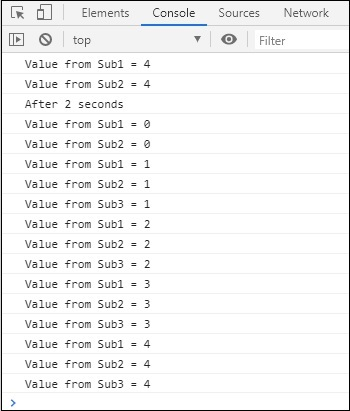# RxJS - Multicasting Operator publishBehavior

publishBehaviour make use of BehaviourSubject, and returns ConnectableObservable. The connect() method has to be used to subscribe to the observable created.

## Syntax

```publishBehaviour(defaultvalue)
```

## Example

```import { interval} from 'rxjs';
import { take, publishBehavior} from 'rxjs/operators';

let observer = interval(1000).pipe(
take(5),
publishBehavior(4)
);
const subscribe_one = observer.subscribe(
x => console.log("Value from Sub1 = "+x)
);
const subscribe_two = observer.subscribe(
x => console.log("Value from Sub2 = "+x)
);
observer.connect();
console.log("After 2 seconds");
setTimeout(() => {
const subscribe_three = observer.subscribe(
x => console.log("Value from Sub3 = "+x)
);
}, 2000);
```

## OutputThe default value will be displayed first and later the value from the observable.